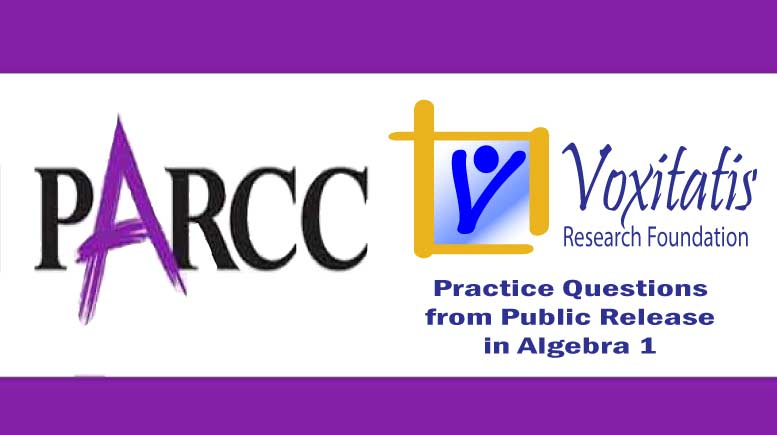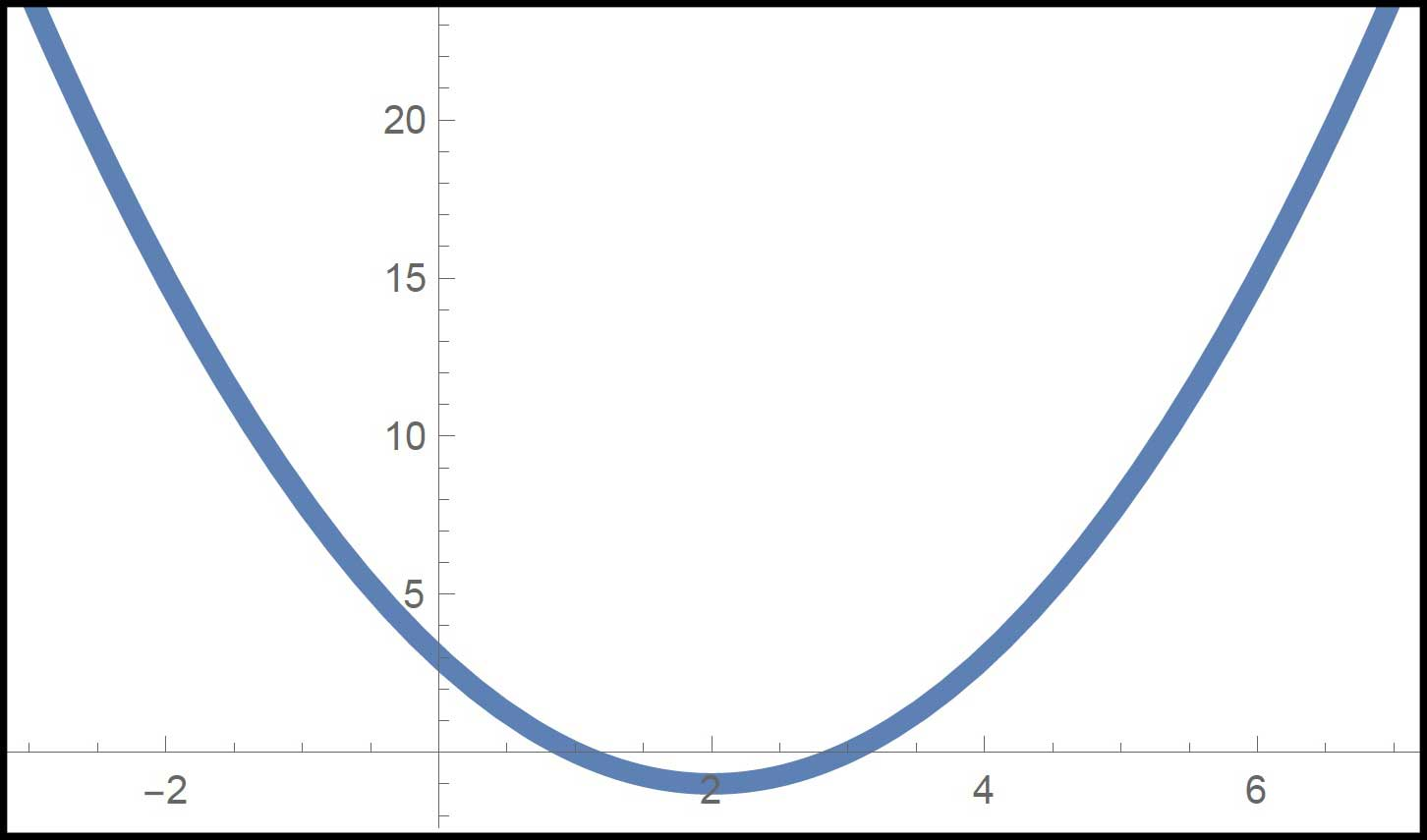Saturday, January 16, 2021# Algebra 1 PARCC question: factor to solve

-#### The following multiple-droplist question, explained here in hopes of helping algebra students in Maryland and Illinois prepare for the PARCC test near the end of this school year, appears on the released version of PARCC’s Spring 2015 test in algebra 1, here:

Select the values and signs from the drop-down menus that correctly complete the solution by factoring.$x^2 - 4x + 3 = 0$

(x _(A)_ _(B)_) (x _(C)_ _(D)_)

x = _(E)_; x = _(F)_

Choices for A and C: + or –
Choices for B and D: 1, 2, 3, or 4
Choices for E: –4, –3, 1, or 2
Choices for F: –2, –1, 3, or 4

Correct answers: A/B: –3, C/D: –1; or A/B: –1, C/D: –3. E/F: 1 and 3.

PARCC evidence statement(s) tested: A-SSE.3a:

Choose and produce an equivalent form of an expression to reveal and explain properties of the quantity represented by the expression. a) Factor a quadratic expression to reveal the zeros of the function it defines.

i) The equivalent form must reveal the zeroes of the function.

ii) Tasks require students to make the connection between the equivalent forms of the expression.

The evidence statement above references Math Practice 7 in the Common Core: Mathematically proficient students look closely to discern a pattern or structure. … Students will see 7 × 8 equals the well remembered 7 × 5 + 7 × 3, in preparation for learning about the distributive property. In the expression x2 + 9x + 14, older students can see the 14 as 2 × 7 and the 9 as 2 + 7. … They also can step back for an overview and shift perspective. They can see complicated things, such as some algebraic expressions, as single objects or as being composed of several objects. For example, they can see 5 – 3(x – y)2 as 5 minus a positive number times a square and use that to realize that its value cannot be more than 5 for any real numbers x and y.

The question tests students’ understanding of the high school Common Core algebra standard HSA.SSE.B.3, found under high school algebra (seeing structure in expressions), which states that they should be able to “choose and produce an equivalent form of an expression to reveal and explain properties of the quantity represented by the expression [and, 3.A] factor a quadratic expression to reveal the zeros of the function it defines.”

Example of a solution strategy (there are others)

Solve a quadratic equation for x by factoring.$x^2 - 4x + 3 = 0$

I can factor the left side of this quadratic equation by noting that

• –1 × –3 = 3
• –1 + –3 = –4$(x-1)(x-3) = 0$

Once we get to this point, we know from the multiplicative property of 0 that if a × b = 0, then either a = 0, b = 0, or both a and b = 0.

So if (x–1) = 0, then x obviously is 1, and if (x–3) = 0, then x must be 3.

All solutions should be checked, which I can do in any number of ways. If I have a graphing calculator (or Mathematica) handy, I can plot the quadratic function on a coordinate grid and see where the graph intersects the x axis. It should be at the solutions I found for x.If I don’t have the means to graph the function, say during the PARCC test, I can plug the values I found for x back into the equation and make sure it’s true.$1^2 - (4)(1) + 3$$1 - 4 + 3 = 0 \quad\textrm{check}$$3^2 - (4)(3) + 3$$9 - 12 + 3 = 0 \quad\textrm{check}$

## Resources for further study

Purple Math, developed by Elizabeth Stapel, a math teacher from the St Louis area, has a six-part series on solving quadratic equations by factoring, as I did above. The series starts here.

The Khan Academy, developed by Sal Khan, an engineer who has created a library of thousands of video lessons, has a series of video lessons that demonstrate how to factor quadratics, starting here. He starts by factoring x2 – 14x + 40 as (x–4)(x–10), even though the text on his site says the second factor is (x–1). Despite the minor error in marketing, which would be unforgivable if done on the PARCC test, the video’s fine. Mr Khan clearly understands completely how to factor quadratics, even if he typed it incorrectly on his site.

Chapter 4, Section 4.4, of the book Algebra 2, Illinois edition by Ron Larson et al deals with solving quadratic equations by factoring. Students are trained to spot the structure in polynomial expressions of the general form$ax^2 + bx + c = (kx+m)(lx+n) = klx^2 + (kn+lm)x + mn$

where k and l must be factors of a and m and n must be factors of c.

Complete reference: Ron Larson, Laurie Boswell, Timothy D Kanold, Lee Stiff. Algebra 2, Illinois edition. Evanston, Ill.: McDougal Littell, a division of Houghton Mifflin Company, 2008. The book is used in several algebra classes taught in Illinois high schools.

## Analysis of this question and online accessibility

The question measures knowledge of the Common Core standard it purports to measure and tests students’ ability, by recognizing structure in an expression, to factor a quadratic equation to find the zeros. It is considered to have a median cognitive demand.

The question can be tested online and should yield results that are as valid and reliable as those obtained on paper. Students online may experience difficulties with the drop-down menus in that the choice the student wishes to select, incorrectly, may not be among the options. Not all possibilities can be made available to students with a question format like this, and not all options for the solutions go with every possible option for the factoring.

No special accommodation challenges can be identified with this question, so the question is considered fair.

## Challenge

What would be a better way to ask this question on a test? Can you identify a context the problem might be useful for?

## Purpose of this series of posts

Voxitatis is developing blog posts that address every algebra 1 question released to the public by the Partnership for Assessment of Readiness for College and Careers, or PARCC, in order to help students prepare to take the test this spring.

Our total release will run from February 27 through March 15, with one or two questions discussed per day. Then we’ll move to geometry at the end of March, algebra 2 during the first half of April, and eighth grade during the last half of April.Paul Katulahttps://news.schoolsdo.org
Paul Katula is the executive editor of the Voxitatis Research Foundation, which publishes this blog. For more information, see the About page.

### Periodic table with superheroes and apps helps

0
Researchers say students retain learning better in chemistry if small modifications are made to the subject's main tool.